Next: 4.1.3 Fourier Analysis of Up: 4.1 Principles of Fourier Previous: 4.1.1 Scalar Diffraction Theory

## 4.1.2 Phase Transformation Properties of a Lens

A thin lens acts as a phase transformation, if a ray entering at coordinates (x, y) on one face emerges at approximately the same coordinates on the opposite face, i.e., if there is negligible translation of the ray within the lens. The phase-change of the ray is given by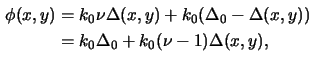withas the refractive index of the material of the lens,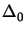as the thickness of the lens on the optical axis, and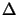(x, y) as the thickness function. The calculation of the thickness function is shown in Figure 4.2,(4.14)

and writes with the paraxial approximation [111, p. 80] to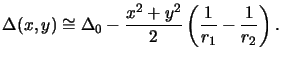(4.15)

The paraxial approximation assumes that the lateral extent (x, y) of the lens is small as compared to the curvature radii r1 and r2, i.e., (x2 + y2)max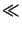r1, r2.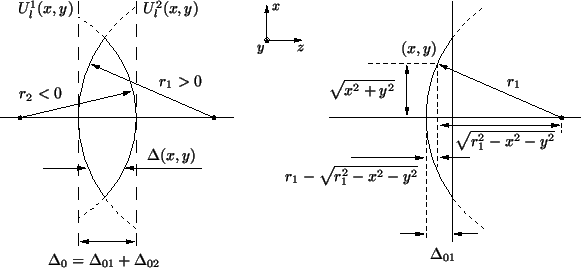The lens transformation is simply given by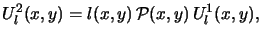(4.16)

where l (x, y) and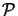(x, y) are the phase transfer function and pupil function of the lens, respectively, and Ul1(x, y) and Ul2(x, y) are the fields entering and emerging the lens. Suppressing the irrelevant constant phase shift k0the phase transfer function follows from (4.16),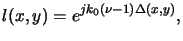(4.17)

and can be simplified with the paraxial approximation (4.18) like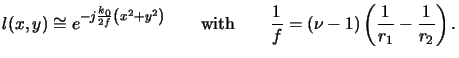(4.18)

Here, f denotes the focal length that combines all physical properties of the lens, i.e., the refractive index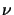and the two curvature radii r1 and r2.

The pupil function(x, y) describes the pass region of the lens. For an ideal diffraction limited circular lens centered on the optical axis(x, y) is given by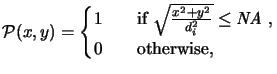(4.19)

where NA is the numerical aperture and di the distance between the image position and the lens plane. The numerical aperture describes the width of the collection cone at the image side and is defined as the sine of the half-collection angle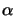, i.e.,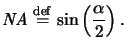(4.20)

A schematic is shown in Figure 4.3. Note that we have defined the numerical aperture at the image side. An analog definition at the object side is possible, but in this case the magnification of the lens has to be considered.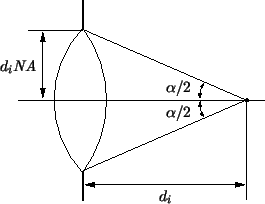Next: 4.1.3 Fourier Analysis of Up: 4.1 Principles of Fourier Previous: 4.1.1 Scalar Diffraction Theory
Heinrich Kirchauer, Institute for Microelectronics, TU Vienna
1998-04-17